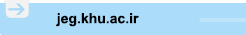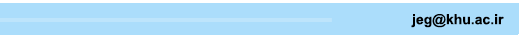[Home ] [Archive]   [ فارسی ]Main About Current Issue Archive Search Submit ContactVolume 13, Issue 2 (Vol. 13, No. 2 2019)2019, 13(2): 371-398 Back to browse issues page
Assessment of Slope Stabilization Based on Probabilistic Analysis Results (Case Study: Slope Facing Spillway of Kavar Dam in Shiraz)
Reza Nassirzadeh Goorchi, Mehdi Amini *1, Hossein Memarian
1- , mamini@ut.ac.ir
Abstract:   (3291 Views)
Introduction
One of the most sensitive and important issues in some civil engineering projects is slope design and application. The process of slope design always involve many uncertainties. Hence, it is impossible to accurately comment on its stability or instability. Most of the uncertainties in the slope stability analysis are related to the nature of materials, geometry, environmental conditions, model errors, and measuring errors as well. Therefore, the slope stability analysis with a deterministic approach which uses the concept of safety factor would often not result satisfactory. Consequently, the use of probabilistic methods is more advised. Accordingly, in recent years, the probability analysis has been used to slope stability analysis. In these analyses, the effective quantities of slope stability are considered as statistical distributions, and the reliability coefficient would then be a statistical distribution. Likewise, one of the approaches to simulate uncertainties in the probabilistic analysis is to use the variation coefficient. If the variation coefficient changes, the probability of failure will change accordingly. When the variation coefficient becomes a larger number, costly solutions are required to reduce the probability of failure. If the variation coefficient becomes low, the reliability will be increased and the required costs to reduce the probability of failure will be decreased. Therefore, determining the amount of variation coefficient in these analyses is very important. Furthermore, the correlation coefficient between the quantities is another effective parameter in computing the probability of failure.
Material and methods
In this research, the stability analysis of the slope facing the spillway of the Shiraz Kavar dam has been done in two probabilistic and deterministic methods. Since circular slip probability is more likely than other types of failure, in the analysis of the stability of this slope, the problem of circular failure is very important, and an appropriate equilibrium program should be used for circular failure analysis. Therefore, SLIDE software was used to slope stability analysis. For material behavior, the Hook-Brown failure criterion was applied. In order to determine the strength parameters of the criterion, Geological Strength Index (GSI), uniaxial compressive strength (UCS) and rock constant parameter mi were used. For crushed rock with a moderate quality of crushing, the GSI quality of the rock mass was about 23 to 38, which the average value of that for the rock mass of the overflow was assumed 35. Also, the uniaxial compressive strength of the rock was evaluated about 50 to 100 MPa with an average value of 75 MPa. In addition, the value of mi was 10, and due to mechanized drilling, the disturbance factor was considered to be 1. The amount of unit weight was assumed to be 22 kN/m3. The initial model used for deterministic and probabilistic analyses, is the Morgenstern-Price model. To conduct probabilistic analyses, Monte Carlo simulation was performed using random sampling method (RS-MC) and 200,000 sampling were used to converge the simulation results. To determine the coefficient of variation and the probability distribution of UCS, GSI and mi, the proposed values ​​of Hook (1998) were applied and for unit weight (γ) James Rodriguez and Sitar (2007) studies were used. Also, the minimum and maximum values ​​of UCS and GSI are determined based on the results of experiments, and Third Sigma rule was utilized for mi and γ quantities. Since the earthquake phenomenon is rarely of great intensity and the number of small earthquakes is higher, therefore the truncated exponential distribution function can be in good agreement with the results of the earthquake. Usually, the maximum magnitude of the earthquake acceleration coefficient is twice that of the average.
Results and discussion
In the presented paper evaluation denotes that the safety factor computed by probabilistic analysis is given as a distribution function. The function provides a clearer view of failure condition. However, a deterministic analysis only illustrates a certain value for the failure. In addition, the results of the probabilistic analysis show that it is possible to optimize the dip of the slope; such that it remains completely stable and the volume of earthwork is also minimized. Therefore, by using probabilistic analysis, the optimal dip of the slope was determined. In these circumstances, the amount of earthwork was decreased by 28,000 cubic meters. Also, the sensitivity analysis of the variation coefficient and correlation coefficient between parameters are analyzed. The results of the sensitivity analysis of the failure probability versus the variation coefficient of the quantities showed that the quantities of sensitivity factor for static conditions is greater than the corresponding pseudo-static, and the GSI amount is the highest, while the specific gravity has the least effect on the probability value. In addition, the analysis indicated that if the GSI coefficient of over 21% is selected, the probability of a static failure is higher than the permissible limit. Also, increasing the variation coefficient of quantities by as much as 50% exhibits that the probability of static failure is still below the permissible limit. Also, the correlation coefficient between UCS and GSI shows that the higher variation coefficient of the quantities is chosen, the more variations of failure probability compared to . In the case of pseudo-static conditions, variations in the failure probability are linear in relation to , while in static conditions, these changes are exponential for an increase of 50% in the variation coefficient. Also, to reduce the coefficient of variation by 50%, the probability of static failure for different values of  is approximately zero.
./files/site1/files/132/8Extended_Abstracts.pdf
Keywords: Probabilistic analysis, Deterministic analysis, Spillway, Shiraz Kavar dam, Sensitivity analysis, Variation coefficient.
Type of Study: Research Paper | Subject: Geotecnic
Received: 2017/04/26 | Accepted: 2018/07/8 | Published: 2018/08/20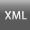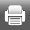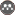Rights and permissionsThis work is licensed under a Creative Commons Attribution-NonCommercial 4.0 International License.